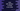# Java program to do left rotation 'n' times to an array### Introduction :

In this tutorial, we will learn how to do the left rotation to an array. We will take the input from the user. The user will input both elements of the array and also the rotation number. Before starting the code, let me show you what is a left rotation and how it looks.

For example, if our main array is [1,2,3,4,5] :

``````start : [1,2,3,4,5]
after first rotation : [2,3,4,5,1]
after second rotation : [3,4,5,1,2]
after third rotation : [4,5,1,2,3]
after fourth rotation : [5,1,2,3,4]
after fifth rotation : [1,2,3,4,5]  ``````

As you can see that the array elements are moved by one position for each rotation.

From the above observation, we can conclude that the 4th element moved to 0th position after 4th rotation, the 0th element moved to the 1st position after 4th rotation etc. Or, one element on current position moved to the (currentposition - n + totalelement)%totalelement_ position, where n is the move number and totalelement_ is the count of the element in the array

Now, let’s try to implement this in java :

### Java Program :

``````import java.util.Arrays;
import java.util.Scanner;

public class Main {
public static void main(String[] args) {
//1
Scanner scanner = new Scanner(System.in);

//2
System.out.println("Enter the count of the array : ");
int count = scanner.nextInt();

System.out.println("Enter the number of rotation : ");
int rotation = scanner.nextInt();

//3
int originalArray[] = new int[count];
int rotatedArray[] = new int[count];

//4
for (int i = 0; i < count; i++) {
System.out.println("Enter element for position " + (i + 1) + " : ");
int no = scanner.nextInt();

//5
originalArray[i] = no;
rotatedArray[(i - rotation + count) % count] = no;
}

//6
System.out.println("Array : " + Arrays.toString(originalArray));
System.out.println("Rotated array : " + Arrays.toString(rotatedArray));
}
}``````

### Explanation :

The commented numbers in the above program denote the step number below :

1. Create one Scanner object to read the user inputs.
2. Ask the user to enter the count of the array and number of rotation. Store these values in count and rotation variables.
3. Create two integer arrays to store the user input and to store the modified array.
4. Run one for loop and read the elements for the array.
5. Store the reading number to the originalArray serially. Also, store the same number in (currentposition - rotationcount + length)%length position.
6. Finally, print out the arrays to the user.

### Sample Output :

``````Enter the count of the array :
5
Enter the number of rotation :
3
Enter element for position 1 :
1
Enter element for position 2 :
2
Enter element for position 3 :
3
Enter element for position 4 :
4
Enter element for position 5 :
5
Array : [1, 2, 3, 4, 5]
Rotated array : [4, 5, 1, 2, 3]``````

### Conclusion :

In this tutorial, we have learnt how to do the left rotation on an array. Try to run the program above and drop a comment below if you have any queries.